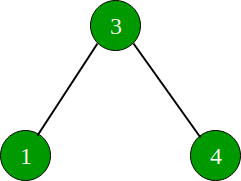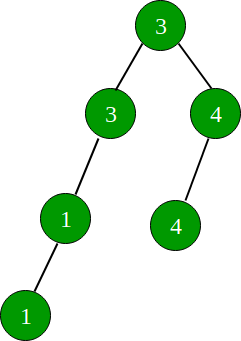Related Articles

# Dailyhunt Interview Experience | Set 1 (On Campus)

• Difficulty Level : Hard
• Last Updated : 07 Feb, 2018

Hello everyone. I am going to share my Dailyhunt interview experience for the profile of Associative Software Engineer. This was an on campus interview.

The process began with elimination round consisting of 5 programing questions mentioned below:

1. You are given N integer numbers (I will define each of them as X here). For all of them, calculate the following:
Sum of all numbers Y such that 1 <= Y < N and GCD(Y, X) has at least two prime factors.
2. Given a string, find the length of the smallest substring consisting of maximum distinct characters.
3. Given a matrix, find the largest rectangular sub-matrix whose sum is 0.
4. Given an array of N integers, form the square matrix of size SQRT(N) x SQRT(N). Rotate this matrix k times. Eventually you will get k + 1 different matrices. You have to multiply all these matrices. Find the sum of the final resulting matrix.
5. Given an array of N integers. Count the number of pairs whose sum exists in the array. E.g. int a[] = {1, 2, 3, 5}. Here there are two such pairs: (1 + 2) = 3, (2 + 3) = 5.

The round was 3 hour long. There were 138 applicants. They shortlisted 22 for the interviews. I had solved 2 questions fully and one partially. I scored 220/550. Exact criteria for the shortlisting is not known but I think they shortlisted students who solved at least two problems completely. There were students with more than 220 marks but they were not selected because they had solved multiple problems partially.

PI round 1 (Technical)
The main aim of this round was to evaluate my basic knowledge of Data Structures, Algorithms and programming language I work upon. I had mentioned java in my resume.

1. Given a linked list, swap the nth node from the beginning with the nth node from the end. You have to swap the nodes itself, not its data.
2. Find the nth largest number from the array.
4. Difference between String and StringBuffer.
5. StringBuffer vs StringBuilder.
6. What is Thread safe and Synchronization?
7. What is serialization?
8. Advantages of StringBuffer and StringBuilder over String.
9. Advantages of Mutable and Immutable Objects.
10. Explain the Interface and its use cases.

The round was approximately 30 minutes long. Then I was told to wait for the next round.

PI round 2 (Technical)

This round had only two main questions and took approximately 30 minutes. It started with my introduction. Then he asked me why Java is so popular and after that we moved on to the main part.

1) Let’s assume user has not performed any activity since last 30 minutes on your website. How would you validate the session? I started with the simple approach we generally follow. We can use session time out and if it is a token based authentication then time out of token. Then he started making the question more complex like this:
Let’s assume server doesn’t support session. You can’t use middleware. Your request should not reach the main class if the session has timed out.

I mentioned my assumption and answered accordingly. I gave him an answer in context of php website. But he asked me to implement the same thing in Servlets. If you know Servlets, you must be familiar with it’s life cycle. My solution failed there. Then he helped me a bit. Told me to implement OOP concept in more detail. Finally, the solution was to use abstract class. If you are familiar with it, you will get the scenario.

2) Given a BST, copy all of its nodes in the same BST.

Initial Tree:Resulting Tree:For all the 3 coding questions, I had to write algorithm pseudocode on the paper.

If you like GeeksforGeeks and would like to contribute, you can also write an article using contribute.geeksforgeeks.org or mail your article to contribute@geeksforgeeks.org. See your article appearing on the GeeksforGeeks main page and help other Geeks.